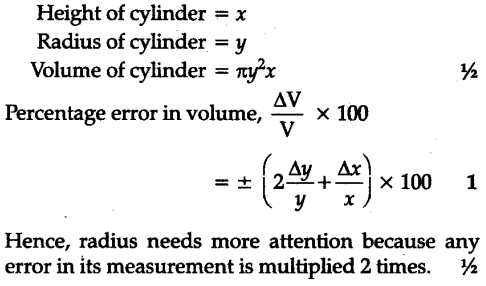# Percentage error is the measurement of height and radius of cylinder are x and y respectively

Percentage error is the measurement of height and radius of cylinder are x and y respectively. Find percentage error in the measurement of volume. Which of the two measurements height or radius need more attention ?